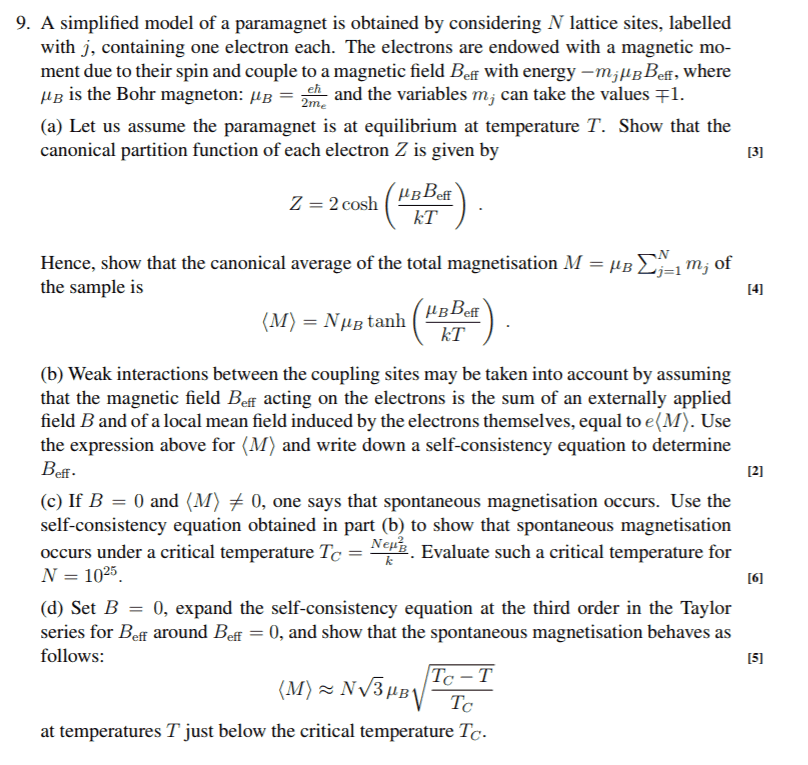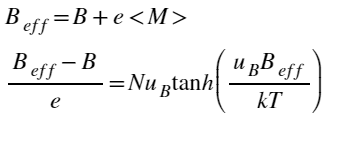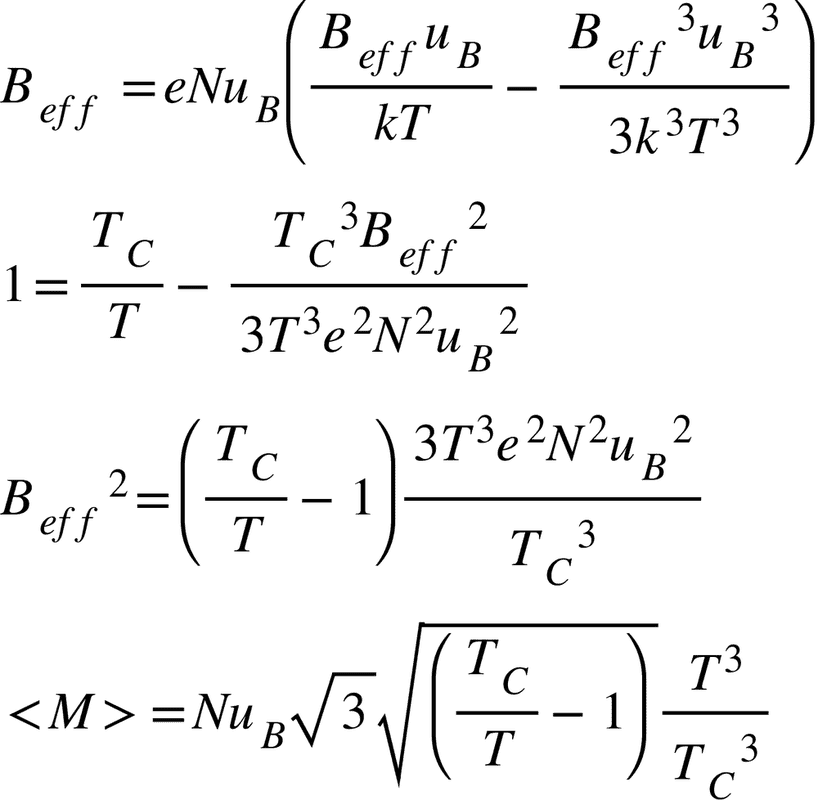# Statistical mechanics problem about a paramagnet

• Clara Chung

#### Clara Chung

Homework Statement
Attached below
Relevant Equations
Attached belowI don't know how to solve part c and d.
Attempt:
c) B_eff=B+e<M>
Substitute T_c into the equation in part b,
=> (B_eff-B)/e = Nμ_B tanh(B_eff/(N*e*μ_B))
Then?
Thank you.

Could you show what you got for part b?

•Clara Chung
Could you show what you got for part b?I just rewrite the equation I found on part A to an equation containing B_eff only... is it what a self-consistency equation mean?...

is it what a self-consistency equation mean?...
A self-consistency equation for ##B_\textrm{eff}## means that you get an equation for ##B_\textrm{eff}## that depends on itself. You could solve it numerically by guessing the value of ##B_\textrm{eff}## in the hyperbolic tangent, then calculating ##B_\textrm{eff}## on the left-hand side, and iterate until the value of ##B_\textrm{eff}## doesn't change anymore.

Your equation for (b) is correct. For (c), I think you can assume that ##B_\textrm{eff}## is small or, more properly
$$\mu_\mathrm{B} B_\textrm{eff} \ll k T$$

•Clara Chung
A self-consistency equation for ##B_\textrm{eff}## means that you get an equation for ##B_\textrm{eff}## that depends on itself. You could solve it numerically by guessing the value of ##B_\textrm{eff}## in the hyperbolic tangent, then calculating ##B_\textrm{eff}## on the left-hand side, and iterate until the value of ##B_\textrm{eff}## doesn't change anymore.

Your equation for (b) is correct. For (c), I think you can assume that ##B_\textrm{eff}## is small or, more properly
$$\mu_\mathrm{B} B_\textrm{eff} \ll k T$$
I did part c using your hintHowever in part d), how to deal with the cube term T^3/T_c^3?

I did part c using your hint
You didn't get the expected answer, which is given in the question. There is no need to keep the cubic terms.

•Clara Chung
You didn't get the expected answer, which is given in the question. There is no need to keep the cubic terms.
Thank you, the answer I displayed above is for part d. The (T/T_C)^3 in the last line should be (T/T_C)^3/2. The expect term have no factor (T/T_C)^3/2, but there is one in my expression...

Above, you wrote
$$B_\textrm{eff} = e N \mu_\mathrm{B} \left( \frac{B_\textrm{eff} \mu_\mathrm{B}}{kT} - \frac{B_\textrm{eff}^3 \mu_\mathrm{B}^3}{3 k^3T^3} \right)$$
which means you took
$$\tanh(x) \approx x - \frac{1}{3} x^3$$

Above, you wrote
$$B_\textrm{eff} = e N \mu_\mathrm{B} \left( \frac{B_\textrm{eff} \mu_\mathrm{B}}{kT} - \frac{B_\textrm{eff}^3 \mu_\mathrm{B}^3}{3 k^3T^3} \right)$$
which means you took
$$\tanh(x) \approx x - \frac{1}{3} x^3$$
•